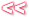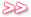Geek Alert : Some basic jacuzzi mathematics18
Aug
'08

It takes one kilo-calorie to heat 1 litre of water by 1 degree C. A calorie is 4.184 joules. In science-speak : “The specific heat capacity of water is 1 cal/gram-C or 4.184 Joule/gram-C”.

A Joule is also a Watt-second. Which relates to the well-known kilowatt-hour by a factor of 3 600 000.

In mathematical terms :

Q = mc(DeltaT) : m = mass, c = specific heat capacity, deltaT = amount you want to heat the water (or anything else) by. The important thing is that all the units match, to get Q in Joule you need c in Joule/gram-C.

The optimal jacuzzi temperature seems to be 39C (102F). So if the water is at 15C, deltaC = 24.

And thumbsuck : the typical jacuzzi is around 1000 litres.

Q = 1000 x 4184 x 24 (Note the sly dropping of the decimal from 4.184 — this is because we’re working with litres not grams, a liter of water weighs 1000 grams) = 100416000 Watt-seconds = 27.89 kWh. Which at the current 47.38c/kWh equates to just over R13.

And with a 4kW element, this will take almost 7 hours. Of course this ignores any losses, anything that’s warm wants to cool down, so it will take more energy and time to actually heat the jacuzzi to 39C.

I’m contemplating keeping the thing at say 25 degrees or so, that won’t lose too much heat to ambient and it’s halfway there, energy and time speaking.